### Probability Mass Function: Binomial Distribution¶

$$\displaystyle f(k,n,p)=\Pr(k;n,p)=\Pr(X=k)={\binom {n}{k}}p^{k}(1-p)^{n-k}$$

for $k = 0, 1, 2, ..., n$.

In [ ]:
#determine distribution
#consider 10 free throw attempts with p = .5
ft = stats.binom(10, 0.5)

In [ ]:
#plot probability
plt.bar(range(11), ft.pmf(range(11)))

Out[ ]:
<BarContainer object of 11 artists>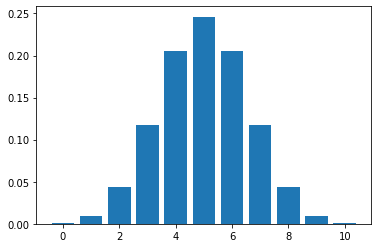In [ ]:
#probability of 6 successes?
ft.pmf(6)

Out[ ]:
0.20507812500000022
In [ ]:
#probability of at least 6 made?
ft.pmf(6) + ft.pmf(7) + ft.pmf(8) + ft.pmf(9) + ft.pmf(10)

Out[ ]:
0.37695312500000033
In [ ]:
#with cumulative distribution function
1 - ft.cdf(5)

Out[ ]:
0.3769531250000001
In [ ]:
#Example 2: p = 0.8, n = 20
ex2 = stats.binom(20, 0.8)

In [ ]:
#P(10)
ex2.pmf(10)

Out[ ]:
0.002031413703013826
In [ ]:
plt.bar(range(21), ex2.pmf(range(21)))

Out[ ]:
<BarContainer object of 21 artists>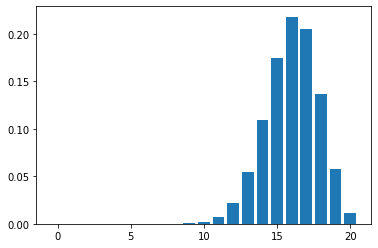In [ ]:
#P(n > 14)
1 - ex2.cdf(13)

Out[ ]:
0.9133074864325998

### Mean and Standard Deviation¶

$$\text{mean} = \mu = \frac{1}{n}\sum_{i = 1}^n x_i$$$$\text{standard deviation} = \sigma = \sqrt{\frac{1}{n}\sum_{i = 1}^n (x_i - \mu)^2}$$
In [ ]:
#mean of a list
x = [3, 5, 7, 9, 11]
np.mean(x)

Out[ ]:
7.0
In [ ]:
#standard deviation of list
np.std(x)

Out[ ]:
2.8284271247461903
In [ ]:
#Binomial distribution with n = 20, p = 0.5
b = stats.binom(20, 0.5)

In [ ]:
#mean
b.mean()

Out[ ]:
10.0
In [ ]:
#plot
plt.bar(range(21), b.pmf(range(21)))

Out[ ]:
<BarContainer object of 21 artists>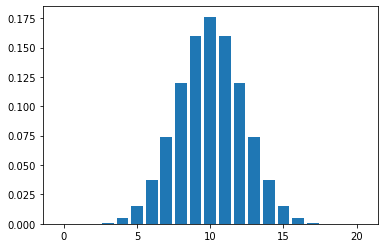In [ ]:
#another example
#Binomial n = 50, p = 0.3
ex2 = stats.binom(50, 0.3)
ex3 = stats.binom(20, 0.3)

In [ ]:
#mean
ex2.mean()

Out[ ]:
15.0
In [ ]:
ex3.mean()

Out[ ]:
6.0
In [ ]:
ex3.std()

Out[ ]:
2.0493901531919194
In [ ]:
#deviation
ex2.std()

Out[ ]:
3.24037034920393
In [ ]:
#plot
plt.bar(range(51), ex2.pmf(range(51)))
plt.bar(range(21), ex3.pmf(range(21)))

Out[ ]:
<BarContainer object of 21 artists>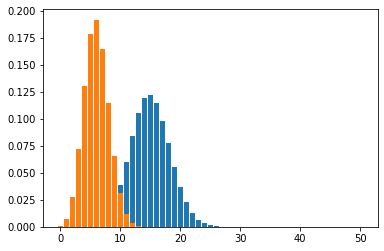### Normal Distribution¶

$$f(x)={\frac {1}{\sigma {\sqrt {2\pi }}}}e^{-{\frac {1}{2}}\left({\frac {x-\mu }{\sigma }}\right)^{2}}}$$
In [ ]:
#determine distribution
##Average height of 60 inches, s.d 2
heights = stats.norm(60, 2)

In [ ]:
#define domain
x = np.linspace(54, 66, 1000)

In [ ]:
#plot distribution
plt.plot(x, heights.pdf(x))

Out[ ]:
[<matplotlib.lines.Line2D at 0x7f0972f418d0>]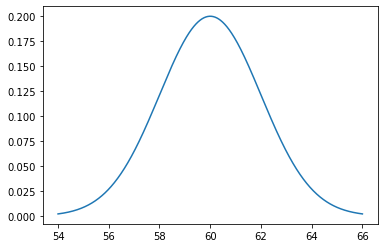In [ ]:
#probability of 65 inches?
heights.pdf(65)

Out[ ]:
0.00876415024678427
In [ ]:
#probability less than 50?
heights.cdf(60)

Out[ ]:
0.5
In [ ]:
heights.mean()

Out[ ]:
60.0
In [ ]:
heights.std()

Out[ ]:
2.0
In [ ]: